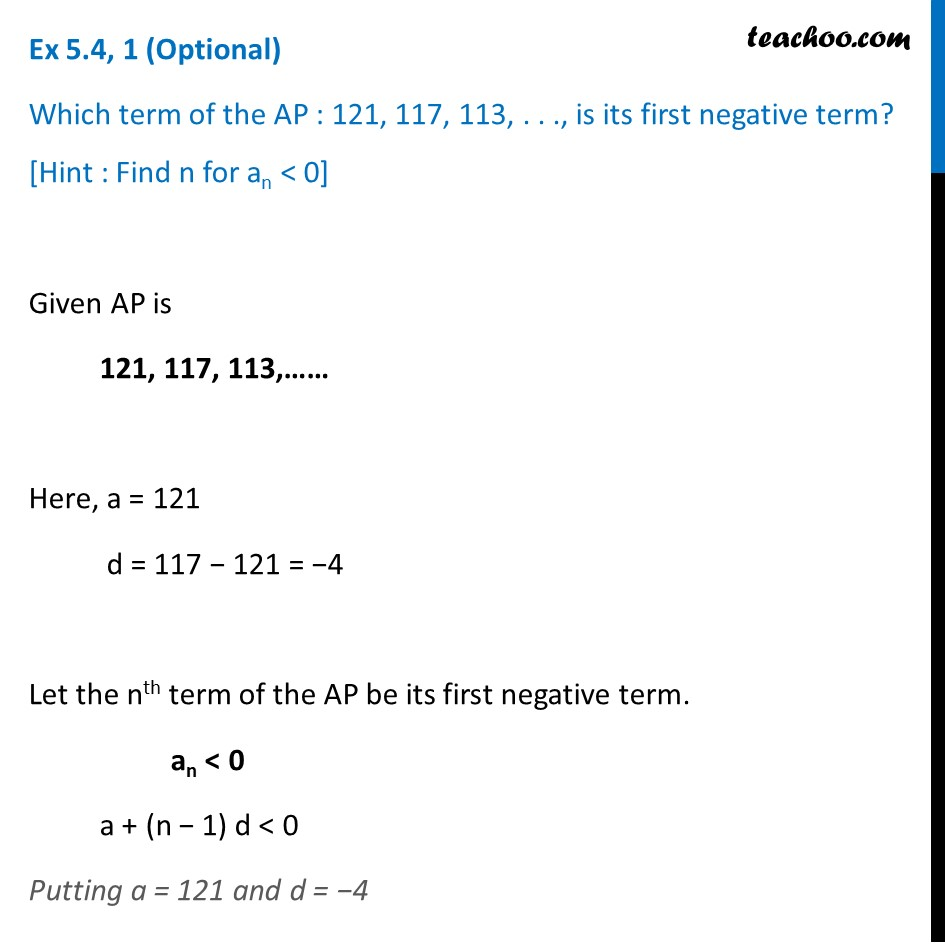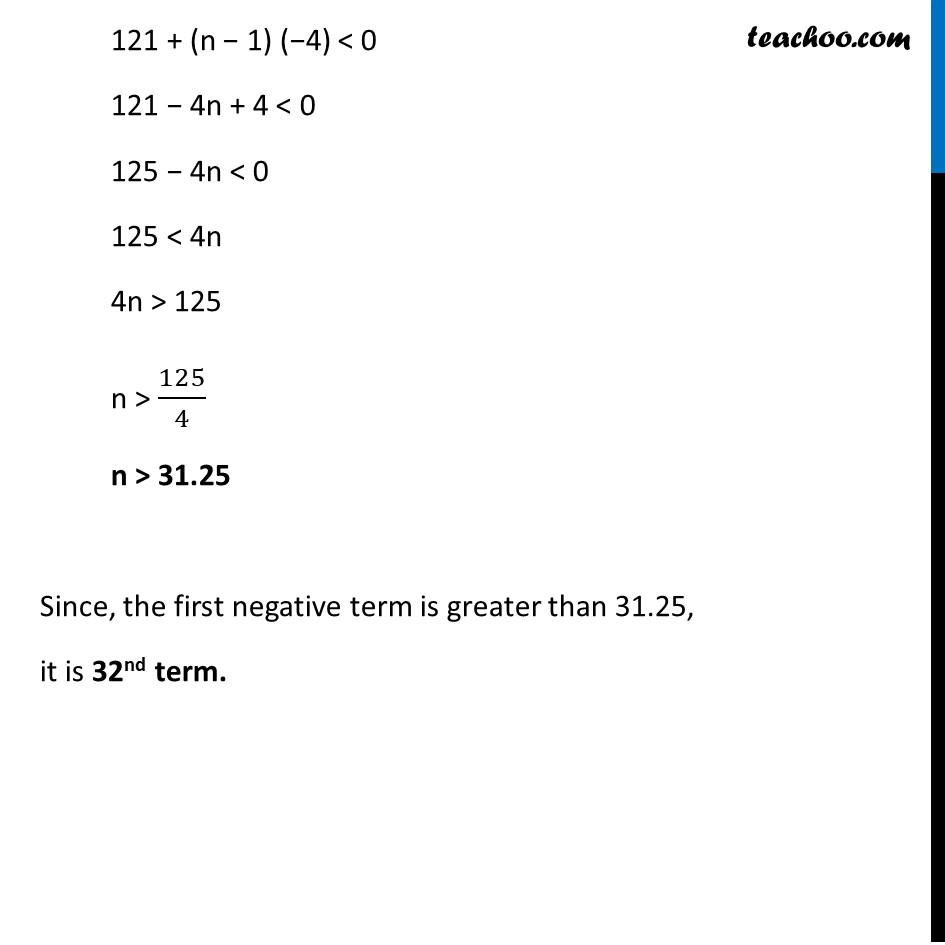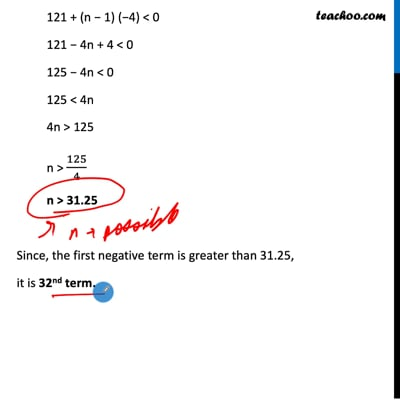Ex 5.4 (Optional)

Chapter 5 Class 10 Arithmetic Progressions
Serial order wiseThis video is only available for Teachoo black users

Maths Crash Course - Live lectures + all videos + Real time Doubt solving!

### Transcript

Ex 5.4, 1 (Optional) Which term of the AP : 121, 117, 113, . . ., is its first negative term? [Hint : Find n for an < 0] Given AP is 121, 117, 113,…… Here, a = 121 d = 117 − 121 = −4 Let the nth term of the AP be its first negative term. an < 0 a + (n − 1) d < 0 Putting a = 121 and d = −4 121 + (n − 1) (−4) < 0 121 − 4n + 4 < 0 125 − 4n < 0 125 < 4n 4n > 125 n > 125/4 n > 31.25 Since, the first negative term is greater than 31.25, it is 32nd term.